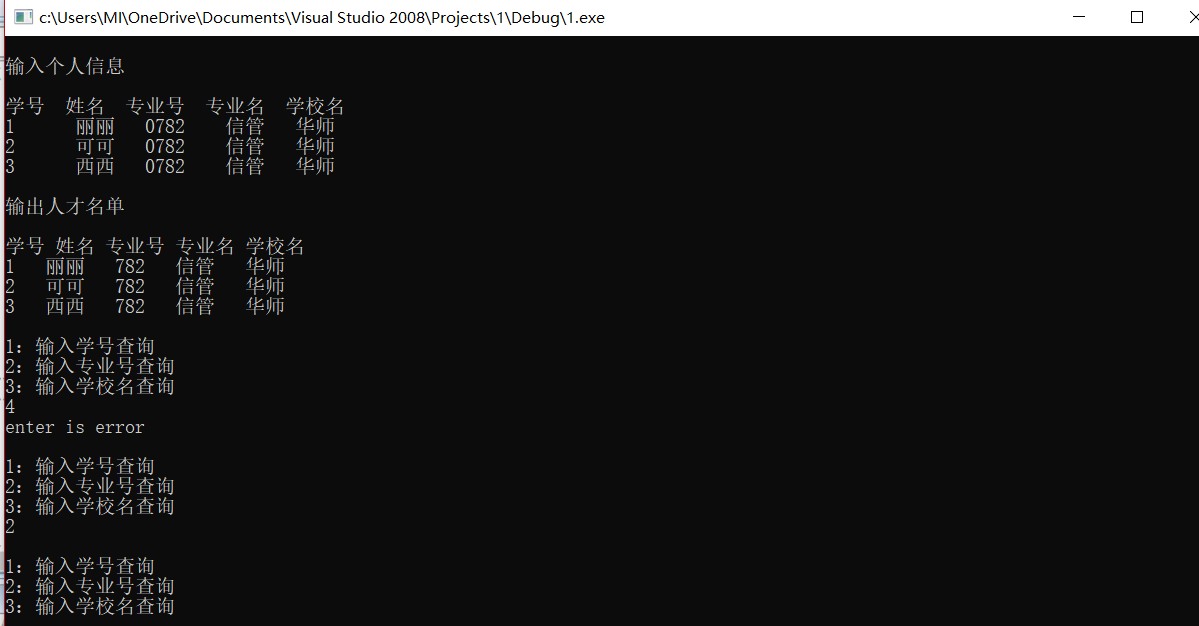C语言新手，写了一段个人信息结构体的代码，但不知道为什么switch调用的函数不能执行。

#include
#include
#define N 3
//建立个人信息结构体
struct STUDNET{
int num;
char name;
int majornum;
char major;
char uni;
}s[N];

//声明查询信息函数
void searchnumber();
void searchmajornum();
void searchuni();

int main(){
int i,j,a;
//输入个人信息
printf("\n输入个人信息\n");
printf("\n学号 姓名 专业号 专业名 学校名\n");
for(i=0;i<N;i++){
scanf("%d%s%d%s%s",&s[i].num,s[i].name,&s[i].majornum,s[i].major,s[i].uni);
}
//输出个人信息
printf("\n输出人才名单\n");
printf("\n学号 姓名 专业号 专业名 学校名\n");
for(j=0;j<N;j++){
printf("%d %s %d %s %s\n",s[j].num,s[j].name,s[j].majornum,s[j].major,s[j].uni);
}
//用循环+选择结构实现不同的查询方式
while("a!=0"){
printf("\n1：输入学号查询\n");
printf("2：输入专业号查询\n");
printf("3：输入学校名查询\n");
scanf("%d",&a);
switch(a){
case 1:void searchnumber();break;//输入学号输出全部信息
case 2:void searchmajornum();break;//输入专业号输出全部信息
case 3:void searchuni();break;//输入学校名输出全部信息
default:printf("enter is error\n");
}
}

}
void searchnumber(){
int b;
int i;
printf("输入学号\n");
scanf("%d",&b);
printf("\n学号 姓名 专业号 专业名 学校名\n");
for(i=0;i<N;i++){
if(b==s[i].num)
printf("%d %s %d %s %s\n",s[i].num,s[i].name,s[i].majornum,s[i].major,s[i].uni);
}
}

void searchmajornum(){
int c;
int i;
printf(" 输入专业号\n");
scanf("%d",&c);
printf("\n学号 姓名 专业号 专业名 学校名\n");
for(i=0;i<N;i++){
if(c==s[i].majornum)
printf("%d %s %d %s %s",s[i].num,s[i].name,s[i].majornum,s[i].major,s[i].uni);
}
}

void searchuni(){
char d;
int i;
printf("输入学校名\n");
scanf("%s",&d);
printf("\n学号 姓名 专业号 专业名 学校名\n");
for(i=0;i<N;i++){
if(strcmp(d,s[i].uni)==0)
printf("%d %s %d %s %s",s[i].num,s[i].name,s[i].majornum,s[i].major,s[i].uni);
}
}

``````
``````5个回答weixin_44023116 我是按照书上的例子写的，不知道为什么会调用不了嘤嘤嘤

case 1:void searchnumber();break;//输入学号输出全部信息

switch里面的函数调用方式有问题，不能加void，直接调用函数名

while("a!=0")？